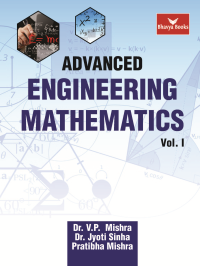•## Advanced Engineering Mathematics Vol. I (Bhavya Books)

In Stock
ISBN 978-93-83992-37-9 Dr. V.P. Mishra, Dr. Jyoti Sinha, Pratibha Mishra Bhavya Books 1st 2018 2019 2018 1000 E-Book In Stock
Rs 695.00Rs 522.00

#### CONTENTS

• Successive Differentiation, Mean Value Theorems and Indeterminate Forms • Applications of Differentiation: Expansion of Functions and Approximations, Curve Tracing, Curvature, Evolutes and Involute, Maxima Minima • Sequences and Series: Convergence and Divergence of Infinite Series, Conditional and Absolute Convergence • Properties of Definite Integrals and Reduction Formulae, Improper Integrals, Beta & Gamma Functions • Applications of Integration—Area, Length, Volume, Surface Area • Multi-variate Differentiation: Partial Differentiation, Euler’s Theorem, Change of Variables • Applications of Multivariate Differentiation: Expansion of Functions of Two Variables, Approximations, Jacobians, Maxima and Minima • Matrices, Determinants, Inverse and Rank of Matrix, Hermitian and Skew-Hermitian Matrices • Solution of System of Equations, Eigen Values, Eigen Vectors, Diagonalizing a Matrix, etc. • Multiple Integration, Double and Triple Integrals and Applications • Fourier Series, Half-Range Series • Vector Differentiation: Gradient, Divergence and Curl • Vector Integration: Line Integral, Surface Integral, Stoke’s Theorem and Gauss’s Theorem • Ordinary Differential Equations of Order One, Linear, Exact, Equations of First Order but not of First Degree, Clairaut’s Equation • Linear Differential Equations of Higher Order, Method of Variation of Parameters and Applications • Series Solution, Bessel’s Differential Equation and Legendre’s Differential Equation • Complex Number System: Complex Functions, Differentiation and Analyticity, Conformal and Bilinear Mapping • Complex Integration: Taylor and Laurent’s Series, Residue Theorem, Evaluation of Real Integrals • Vector Spaces • Basic Statistics • Applied Statistics – Curve Fitting, Correlation and Regression • Theory of Probability • Discrete Probability Distributions • Continuous Probability Distributions • Sampling, Tests of Significance for Large Samples • Tests for Small Samples • Answers and Hints to the Problems in Exercises.

• Author : Dr. V.P. Mishra, Dr. Jyoti Sinha, Pratibha Mishra
• Publisher : Bhavya Books
• Edition : 1st 2018
• Reprint : 2019
• Publishing Year : 2018
• Total Pages : 1000

## My Wish List

Please login to access Wishltist

Economy Delivery(Print Book Only):
in 7 - 10 Working Days.

Express Delivery(Print Book Only) :
in 5 -7 Working Days

Subject to availability.